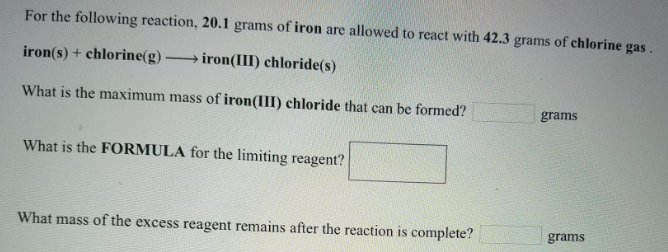# Problem: For the following reaction, 20.1 grams of iron are allowed to react with 42.3 grams of chlorine gas.iron (s) + chlorine (g) → iron(III) chloride(s) What is the maximum mass of iron(III) chloride that can be formed? What is the FORMULA for the limiting reagent? What mass of the excess reagent remains after the reaction is complete?

###### FREE Expert Solution
95% (307 ratings)###### Problem Details

For the following reaction, 20.1 grams of iron are allowed to react with 42.3 grams of chlorine gas.

iron (s) + chlorine (g) → iron(III) chloride(s)

What is the maximum mass of iron(III) chloride that can be formed?

What is the FORMULA for the limiting reagent?

What mass of the excess reagent remains after the reaction is complete?